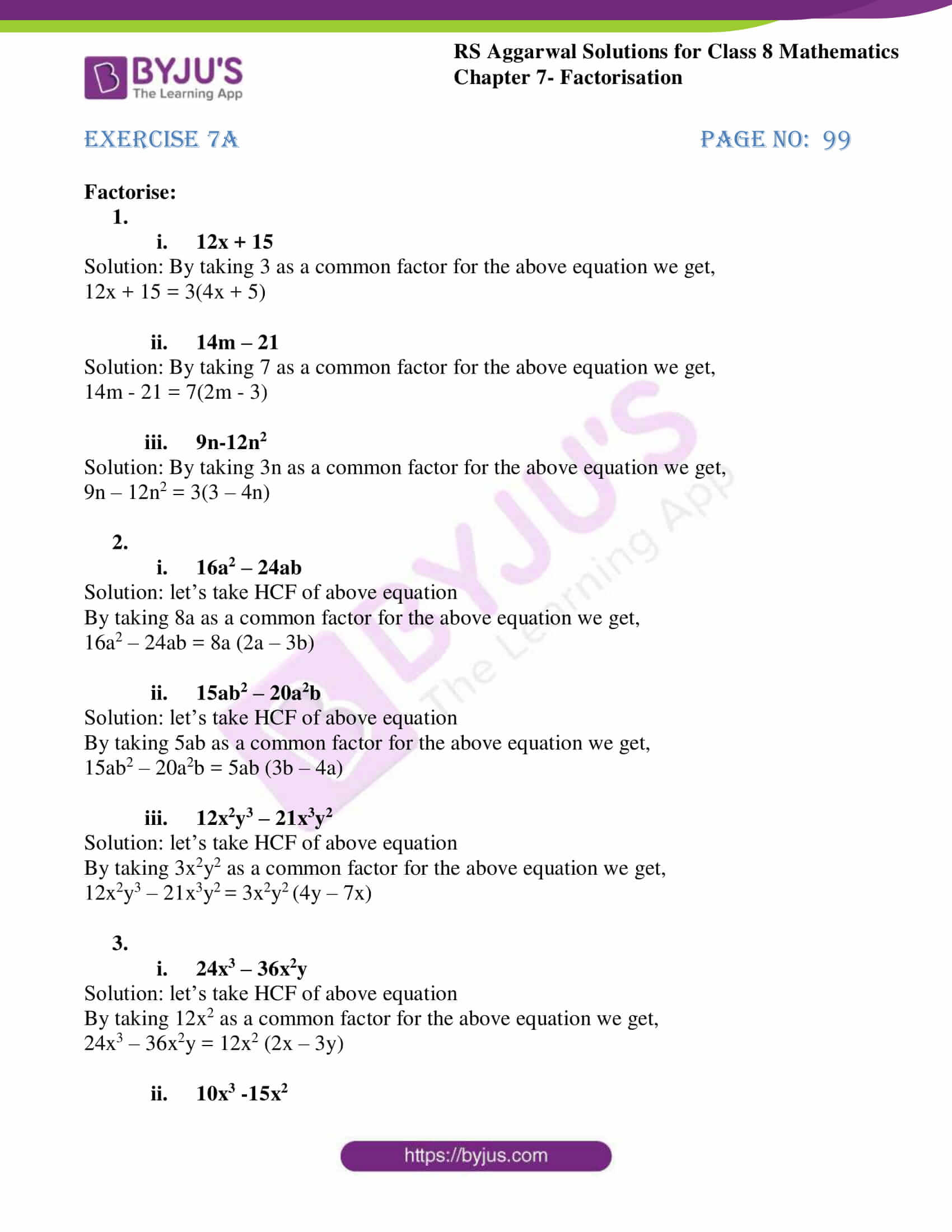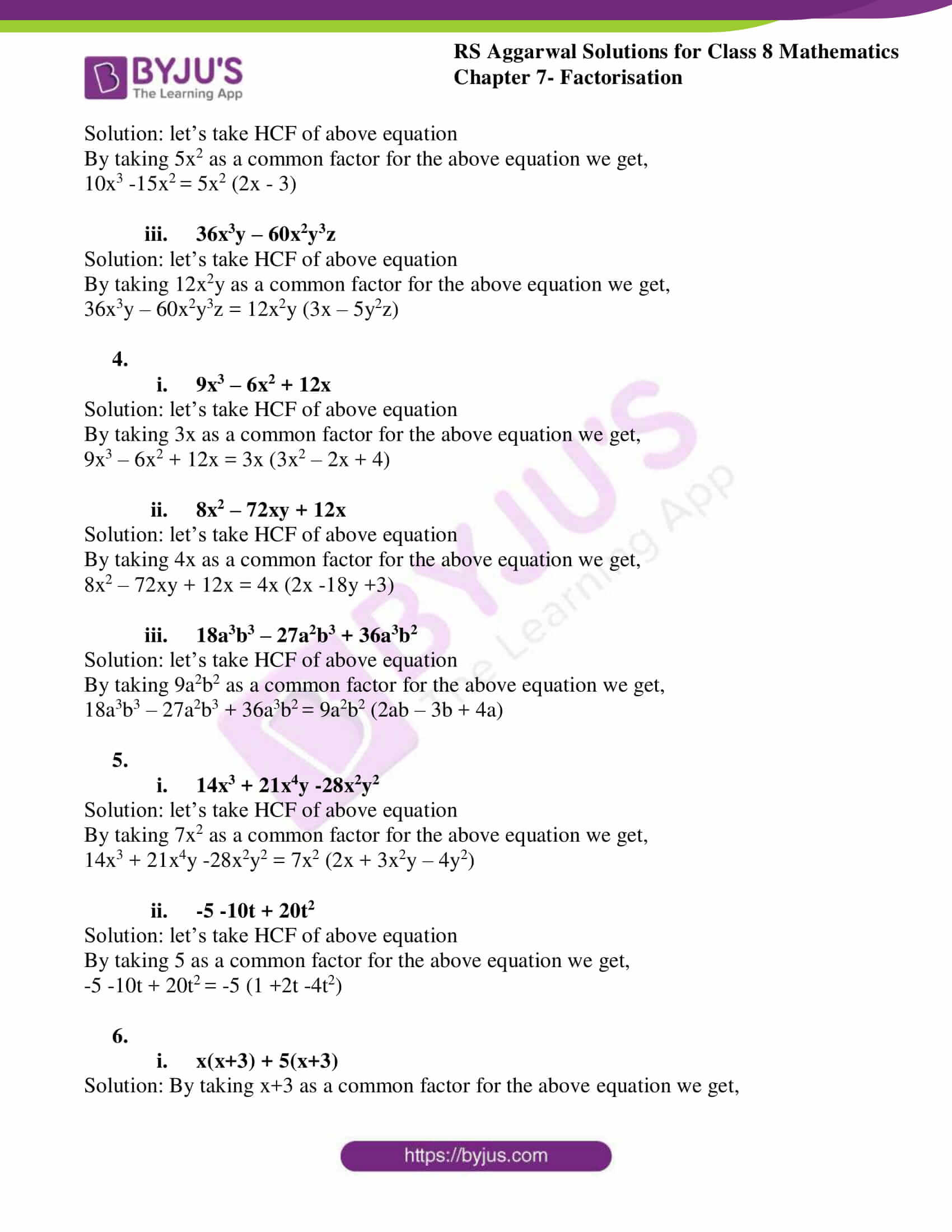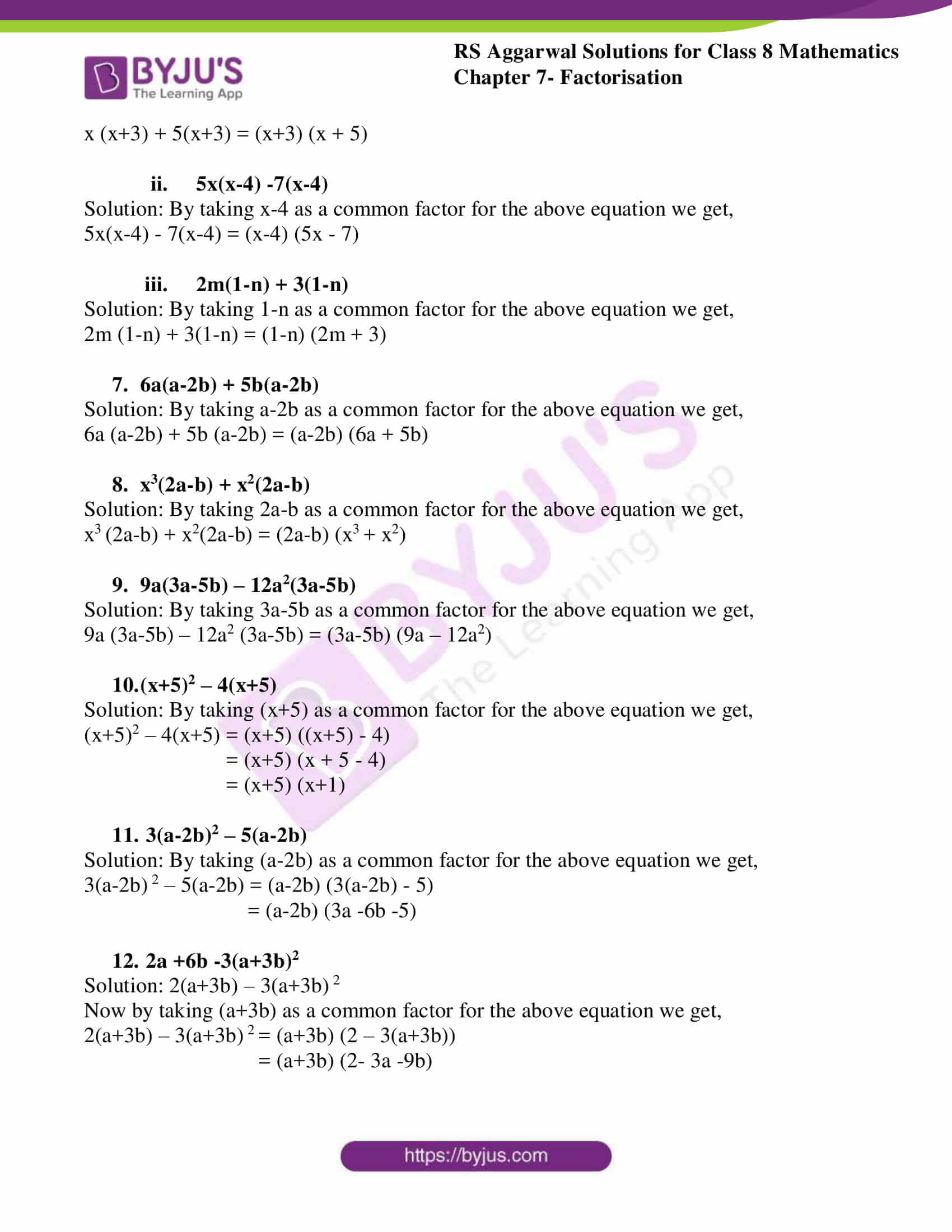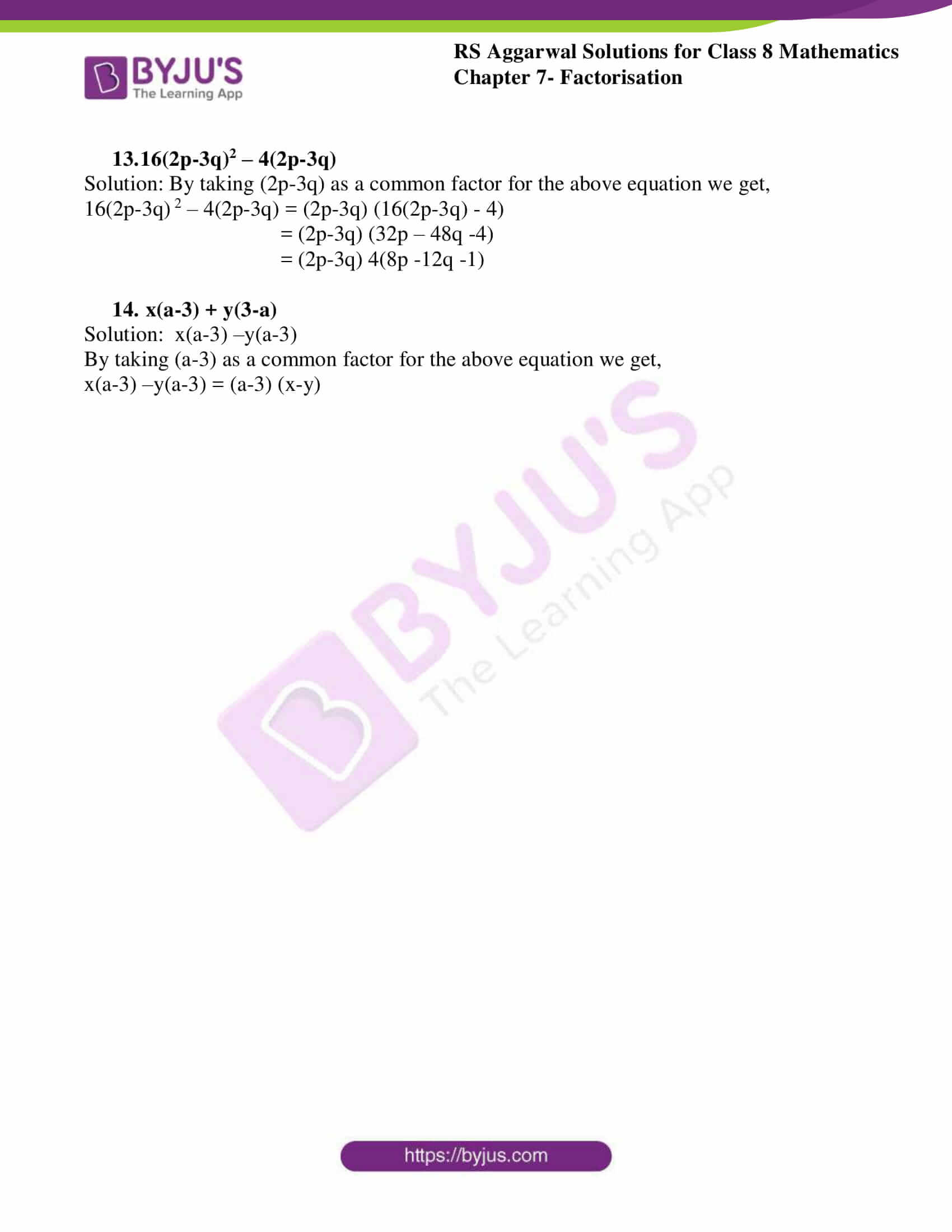# RS Aggarwal Solutions for Class 8 Chapter 7 - Factorisation Exercise 7A

Our expert team has solved the RS Aggarwal Solutions to ensure that the students are thorough with their fundamentals by practicing the solutions. Students can refer and download RS Aggarwal Solutions for Class 8 Maths Chapter 7- Exercise 7A, Factorisation from the links provided below.

In Exercise 7A of RS Aggarwal Class 8 Maths, we shall study about Factors and Factorisation. What are Factors? When an algebraic expression can be written as the product of two or more expressions, then each of these expressions is called the factor of the given expression. What is Factorisation? Factorisation is the reverse process of multiplication.

## Download PDF of RS Aggarwal Solutions for Class 8 Maths Chapter 7- Exercise 7A### Access Answers to RS Aggarwal Solutions for Class 8 Maths Chapter 7- Factorisation Exercise 7A

Factorise:

1.

1. 12x + 15

Solution: By taking 3 as a common factor for the above equation we get,

12x + 15 = 3(4x + 5)

2. 14m – 21

Solution: By taking 7 as a common factor for the above equation we get,

14m – 21 = 7(2m – 3)

3. 9n-12n2

Solution: By taking 3n as a common factor for the above equation we get,

9n – 12n2 = 3(3 – 4n)

2.

1. 16a2 – 24ab

Solution: let’s take HCF of above equation

By taking 8a as a common factor for the above equation we get,

16a2 – 24ab = 8a (2a – 3b)

2. 15ab2 – 20a2b

Solution: let’s take HCF of above equation

By taking 5ab as a common factor for the above equation we get,

15ab2 – 20a2b = 5ab (3b – 4a)

3. 12x2y3 – 21x3y2

Solution: let’s take HCF of above equation

By taking 3x2y2 as a common factor for the above equation we get,

12x2y3 – 21x3y2 = 3x2y2 (4y – 7x)

3.

1. 24x3 – 36x2y

Solution: let’s take HCF of above equation

By taking 12x2 as a common factor for the above equation we get,

24x3 – 36x2y = 12x2 (2x – 3y)

2. 10x3 -15x2

Solution: let’s take HCF of above equation

By taking 5x2 as a common factor for the above equation we get,

10x3 -15x2 = 5x2 (2x – 3)

3. 36x3y – 60x2y3z

Solution: let’s take HCF of above equation

By taking 12x2y as a common factor for the above equation we get,

36x3y – 60x2y3z = 12x2y (3x – 5y2z)

4.

1. 9x3 – 6x2 + 12x

Solution: let’s take HCF of above equation

By taking 3x as a common factor for the above equation we get,

9x3 – 6x2 + 12x = 3x (3x2 – 2x + 4)

2. 8x2 – 72xy + 12x

Solution: let’s take HCF of above equation

By taking 4x as a common factor for the above equation we get,

8x2 – 72xy + 12x = 4x (2x -18y +3)

3. 18a3b3 – 27a2b3 + 36a3b2

Solution: let’s take HCF of above equation

By taking 9a2b2 as a common factor for the above equation we get,

18a3b3 – 27a2b3 + 36a3b2 = 9a2b2 (2ab – 3b + 4a)

5.

1. 14x3 + 21x4y -28x2y2

Solution: let’s take HCF of above equation

By taking 7x2 as a common factor for the above equation we get,

14x3 + 21x4y -28x2y2 = 7x2 (2x + 3x2y – 4y2)

2. -5 -10t + 20t2

Solution: let’s take HCF of above equation

By taking 5 as a common factor for the above equation we get,

-5 -10t + 20t2 = -5 (1 +2t -4t2)

6.

1. x(x+3) + 5(x+3)

Solution: By taking x+3 as a common factor for the above equation we get,

x (x+3) + 5(x+3) = (x+3) (x + 5)

2. 5x(x-4) -7(x-4)

Solution: By taking x-4 as a common factor for the above equation we get,

5x(x-4) – 7(x-4) = (x-4) (5x – 7)

3. 2m(1-n) + 3(1-n)

Solution: By taking 1-n as a common factor for the above equation we get,

2m (1-n) + 3(1-n) = (1-n) (2m + 3)

7. 6a(a-2b) + 5b(a-2b)

Solution: By taking a-2b as a common factor for the above equation we get,

6a (a-2b) + 5b (a-2b) = (a-2b) (6a + 5b)

8. x3(2a-b) + x2(2a-b)

Solution: By taking 2a-b as a common factor for the above equation we get,

x3 (2a-b) + x2(2a-b) = (2a-b) (x3 + x2)

9. 9a(3a-5b) – 12a2(3a-5b)

Solution: By taking 3a-5b as a common factor for the above equation we get,

9a (3a-5b) – 12a2 (3a-5b) = (3a-5b) (9a – 12a2)

10. (x+5)2 – 4(x+5)

Solution: By taking (x+5) as a common factor for the above equation we get,

(x+5)2 – 4(x+5) = (x+5) ((x+5) – 4)

= (x+5) (x + 5 – 4)

= (x+5) (x+1)

11. 3(a-2b)2 – 5(a-2b)

Solution: By taking (a-2b) as a common factor for the above equation we get,

3(a-2b) 2 – 5(a-2b) = (a-2b) (3(a-2b) – 5)

= (a-2b) (3a -6b -5)

12. 2a +6b -3(a+3b)2

Solution: 2(a+3b) – 3(a+3b) 2

Now by taking (a+3b) as a common factor for the above equation we get,

2(a+3b) – 3(a+3b) 2 = (a+3b) (2 – 3(a+3b))

= (a+3b) (2- 3a -9b)

13. 16(2p-3q)2 – 4(2p-3q)

Solution: By taking (2p-3q) as a common factor for the above equation we get,

16(2p-3q) 2 – 4(2p-3q) = (2p-3q) (16(2p-3q) – 4)

= (2p-3q) (32p – 48q -4)

= (2p-3q) 4(8p -12q -1)

14. x(a-3) + y(3-a)

Solution: x(a-3) –y(a-3)

By taking (a-3) as a common factor for the above equation we get,

x(a-3) –y(a-3) = (a-3) (x-y)

## RS Aggarwal Solutions for Class 8 Maths Chapter 7- Exercise 7A

Exercise 7A of RS Aggarwal Class 8, Factorisation. This exercise mainly deals with Factors and Factorisation by different methods i.e.

• Factorisation when a common monomial factor occurs in each term.
• Factorisation when a binomial is common.
• Factorisation by grouping.

The RS Aggarwal Solutions can help the students practice diligently while learning the fundamentals as it provides all the answers to the questions from the RS Aggarwal textbook. Students are suggested to practice the problems very sincerely, which would help them excel in their exams and increase their overall percentage. Practicing as many times as possible helps accelerating time management abilities and also boosts the confidence level to achieve high marks.